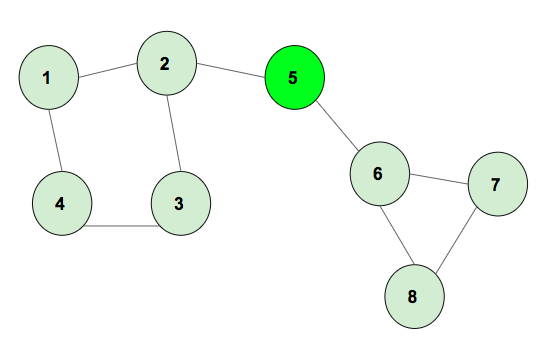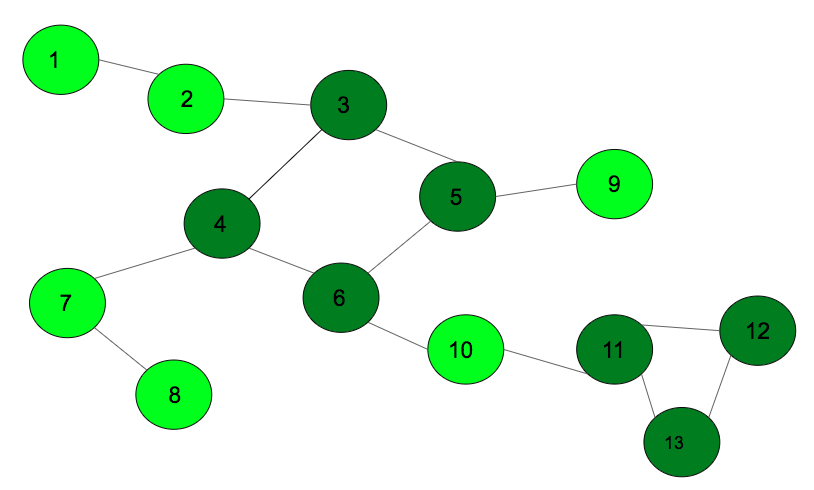# Product of lengths of all cycles in an undirected graph

• Difficulty Level : Hard
• Last Updated : 01 Sep, 2022

Given an undirected and unweighted graph. The task is to find the product of the lengths of all cycles formed in it.
Example 1:The above graph has two cycles of length 4 and 3, the product of cycle lengths is 12.

Example 2:The above graph has two cycles of length 4 and 3, the product of cycle lengths is 12.

Approach: Using the graph coloring method, mark all the vertex of the different cycles with unique numbers. Once the graph traversal is completed, push all the similar marked numbers to an adjacency list and print the adjacency list accordingly.

Given below is the algorithm:

• Insert the edges into an adjacency list.
• Call the DFS function which uses the coloring method to mark the vertex.
• Whenever there is a partially visited vertex, backtrack till the current vertex is reached and mark all of them with cycle numbers. Once all the vertexes are marked, increase the cycle number.
• Once Dfs is completed, iterate for the edges and push the same marked number edges to another adjacency list.
• Iterate in the another adjacency list, and keep the count of number of vertex in a cycle using map and cycle numbers
• Iterate for cycle numbers, and multiply the lengths to get the final product which will be the answer.

Below is the implementation of the above approach:

## C++

 `// C++ program to find the``// product of lengths of cycle``#include ``using` `namespace` `std;``const` `int` `N = 100000;` `// variables to be used``// in both functions``vector<``int``> graph[N];` `// Function to mark the vertex with``// different colors for different cycles``void` `dfs_cycle(``int` `u, ``int` `p, ``int` `color[],``            ``int` `mark[], ``int` `par[], ``int``& cyclenumber)``{` `    ``// already (completely) visited vertex.``    ``if` `(color[u] == 2) {``        ``return``;``    ``}` `    ``// seen vertex, but was not completely``    ``// visited -> cycle detected.``    ``// backtrack based on parents to find``    ``// the complete cycle.``    ``if` `(color[u] == 1) {` `        ``cyclenumber++;``        ``int` `cur = p;``        ``mark[cur] = cyclenumber;` `        ``// backtrack the vertex which are``        ``// in the current cycle thats found``        ``while` `(cur != u) {``            ``cur = par[cur];``            ``mark[cur] = cyclenumber;``        ``}``        ``return``;``    ``}``    ``par[u] = p;` `    ``// partially visited.``    ``color[u] = 1;` `    ``// simple dfs on graph``    ``for` `(``int` `v : graph[u]) {` `        ``// if it has not been visited previously``        ``if` `(v == par[u]) {``            ``continue``;``        ``}``        ``dfs_cycle(v, u, color, mark, par, cyclenumber);``    ``}` `    ``// completely visited.``    ``color[u] = 2;``}` `// add the edges to the graph``void` `addEdge(``int` `u, ``int` `v)``{``    ``graph[u].push_back(v);``    ``graph[v].push_back(u);``}` `// Function to print the cycles``int` `productLength(``int` `edges, ``int` `mark[], ``int``& cyclenumber)``{``    ``unordered_map<``int``, ``int``> mp;` `    ``// push the edges that into the``    ``// cycle adjacency list``    ``for` `(``int` `i = 1; i <= edges; i++) {``        ``if` `(mark[i] != 0)``            ``mp[mark[i]]++;``    ``}``    ``int` `cnt = 1;` `    ``// product all the length of cycles``    ``for` `(``int` `i = 1; i <= cyclenumber; i++) {``        ``cnt = cnt * mp[i];``    ``}``    ``if` `(cyclenumber == 0)``        ``cnt = 0;` `    ``return` `cnt;``}` `// Driver Code``int` `main()``{` `    ``// add edges``    ``addEdge(1, 2);``    ``addEdge(2, 3);``    ``addEdge(3, 4);``    ``addEdge(4, 6);``    ``addEdge(4, 7);``    ``addEdge(5, 6);``    ``addEdge(3, 5);``    ``addEdge(7, 8);``    ``addEdge(6, 10);``    ``addEdge(5, 9);``    ``addEdge(10, 11);``    ``addEdge(11, 12);``    ``addEdge(11, 13);``    ``addEdge(12, 13);` `    ``// arrays required to color the``    ``// graph, store the parent of node``    ``int` `color[N];``    ``int` `par[N];` `    ``// mark with unique numbers``    ``int` `mark[N];` `    ``// store the numbers of cycle``    ``int` `cyclenumber = 0;``    ``int` `edges = 13;` `    ``// call DFS to mark the cycles``    ``dfs_cycle(1, 0, color, mark, par, cyclenumber);` `    ``// function to print the cycles``    ``cout << productLength(edges, mark, cyclenumber);` `    ``return` `0;``}`

## Java

 `// Java program to find the``// product of lengths of cycle``import` `java.io.*;``import` `java.util.*;` `class` `GFG``{``    ``static` `int` `N = ``100000``;``    ``static` `int` `cyclenumber;` `    ``// variables to be used``    ``// in both functions``    ``//@SuppressWarnings("unchecked")``    ``static` `Vector[] graph = ``new` `Vector[N];` `    ``// This static block is used to initialize``    ``// array of Vector, otherwise it will throw``    ``// NullPointerException``    ``static``    ``{``        ``for` `(``int` `i = ``0``; i < N; i++)``            ``graph[i] = ``new` `Vector<>();``    ``}` `    ``// Function to mark the vertex with``    ``// different colors for different cycles``    ``static` `void` `dfs_cycle(``int` `u, ``int` `p, ``int``[] color,``                          ``int``[] mark, ``int``[] par)``    ``{` `        ``// already (completely) visited vertex.``        ``if` `(color[u] == ``2``)``            ``return``;` `        ``// seen vertex, but was not completely``        ``// visited -> cycle detected.``        ``// backtrack based on parents to find``        ``// the complete cycle.``        ``if` `(color[u] == ``1``)``        ``{``            ``cyclenumber++;``            ``int` `cur = p;``            ``mark[cur] = cyclenumber;` `            ``// backtrack the vertex which are``            ``// in the current cycle thats found``            ``while` `(cur != u)``            ``{``                ``cur = par[cur];``                ``mark[cur] = cyclenumber;``            ``}``            ``return``;``        ``}``        ``par[u] = p;` `        ``// partially visited.``        ``color[u] = ``1``;` `        ``// simple dfs on graph``        ``for` `(``int` `v : graph[u])``        ``{` `            ``// if it has not been visited previously``            ``if` `(v == par[u])``            ``{``                ``continue``;``            ``}``            ``dfs_cycle(v, u, color, mark, par);``        ``}` `        ``// completely visited.``        ``color[u] = ``2``;``    ``}` `    ``// add the edges to the graph``    ``static` `void` `addEdge(``int` `u, ``int` `v)``    ``{``        ``graph[u].add(v);``        ``graph[v].add(u);``    ``}` `    ``// Function to print the cycles``    ``static` `int` `productLength(``int` `edges, ``int``[] mark)``    ``{``        ``HashMap mp = ``new` `HashMap<>();` `        ``// push the edges that into the``        ``// cycle adjacency list``        ``for` `(``int` `i = ``1``; i <= edges; i++)``        ``{``            ``if` `(mark[i] != ``0``)``            ``{``                ``mp.put(mark[i], mp.get(mark[i]) == ``null` `?``                                ``1` `: mp.get(mark[i]) + ``1``);``            ``}``        ``}``        ``int` `cnt = ``1``;` `        ``// product all the length of cycles``        ``for` `(``int` `i = ``1``; i <= cyclenumber; i++)``        ``{``            ``cnt = cnt * mp.get(i);``        ``}``        ``if` `(cyclenumber == ``0``)``            ``cnt = ``0``;` `        ``return` `cnt;``    ``}` `    ``// Driver Code``    ``public` `static` `void` `main(String[] args) ``throws` `IOException``    ``{` `        ``// add edges``        ``addEdge(``1``, ``2``);``        ``addEdge(``2``, ``3``);``        ``addEdge(``3``, ``4``);``        ``addEdge(``4``, ``6``);``        ``addEdge(``4``, ``7``);``        ``addEdge(``5``, ``6``);``        ``addEdge(``3``, ``5``);``        ``addEdge(``7``, ``8``);``        ``addEdge(``6``, ``10``);``        ``addEdge(``5``, ``9``);``        ``addEdge(``10``, ``11``);``        ``addEdge(``11``, ``12``);``        ``addEdge(``11``, ``13``);``        ``addEdge(``12``, ``13``);` `        ``// arrays required to color the``        ``// graph, store the parent of node``        ``int``[] color = ``new` `int``[N];``        ``int``[] par = ``new` `int``[N];` `        ``// mark with unique numbers``        ``int``[] mark = ``new` `int``[N];` `        ``// store the numbers of cycle``        ``cyclenumber = ``0``;``        ``int` `edges = ``13``;` `        ``// call DFS to mark the cycles``        ``dfs_cycle(``1``, ``0``, color, mark, par);` `        ``// function to print the cycles``        ``System.out.println(productLength(edges, mark));``    ``}``}` `// This code is contributed by``// sanjeev2552`

## Python3

 `# Python3 program to find the``# product of lengths of cycle``from` `collections ``import` `defaultdict` `# Function to mark the vertex with``# different colors for different cycles``def` `dfs_cycle(u, p, color, mark, par):` `    ``global` `cyclenumber``    ` `    ``# already (completely) visited vertex.``    ``if` `color[u] ``=``=` `2``:``        ``return` `    ``# seen vertex, but was not completely``    ``# visited -> cycle detected.``    ``# backtrack based on parents to find``    ``# the complete cycle.``    ``if` `color[u] ``=``=` `1``:` `        ``cyclenumber ``+``=` `1``        ``cur ``=` `p``        ``mark[cur] ``=` `cyclenumber` `        ``# backtrack the vertex which are``        ``# in the current cycle thats found``        ``while` `cur !``=` `u:``            ``cur ``=` `par[cur]``            ``mark[cur] ``=` `cyclenumber``        ` `        ``return``    ` `    ``par[u] ``=` `p` `    ``# partially visited.``    ``color[u] ``=` `1` `    ``# simple dfs on graph``    ``for` `v ``in` `graph[u]:` `        ``# if it has not been visited previously``        ``if` `v ``=``=` `par[u]:``            ``continue``        ` `        ``dfs_cycle(v, u, color, mark, par)``    ` `    ``# completely visited.``    ``color[u] ``=` `2` `# add the edges to the graph``def` `addEdge(u, v):` `    ``graph[u].append(v)``    ``graph[v].append(u)` `# Function to print the cycles``def` `productLength(edges, mark, cyclenumber):` `    ``mp ``=` `defaultdict(``lambda``:``0``)` `    ``# push the edges that into the``    ``# cycle adjacency list``    ``for` `i ``in` `range``(``1``, edges``+``1``):``        ``if` `mark[i] !``=` `0``:``            ``mp[mark[i]] ``+``=` `1``    ` `    ``cnt ``=` `1` `    ``# product all the length of cycles``    ``for` `i ``in` `range``(``1``, cyclenumber ``+` `1``):``        ``cnt ``=` `cnt ``*` `mp[i]``    ` `    ``if` `cyclenumber ``=``=` `0``:``        ``cnt ``=` `0` `    ``return` `cnt` `# Driver Code``if` `__name__ ``=``=` `"__main__"``:``    ` `    ``N ``=` `100000``    ``graph ``=` `[[] ``for` `i ``in` `range``(N)]` `    ``# add edges``    ``addEdge(``1``, ``2``)``    ``addEdge(``2``, ``3``)``    ``addEdge(``3``, ``4``)``    ``addEdge(``4``, ``6``)``    ``addEdge(``4``, ``7``)``    ``addEdge(``5``, ``6``)``    ``addEdge(``3``, ``5``)``    ``addEdge(``7``, ``8``)``    ``addEdge(``6``, ``10``)``    ``addEdge(``5``, ``9``)``    ``addEdge(``10``, ``11``)``    ``addEdge(``11``, ``12``)``    ``addEdge(``11``, ``13``)``    ``addEdge(``12``, ``13``)` `    ``# arrays required to color the``    ``# graph, store the parent of node``    ``color, par ``=` `[``None``] ``*` `N, [``None``] ``*` `N` `    ``# mark with unique numbers``    ``mark ``=` `[``None``] ``*` `N` `    ``# store the numbers of cycle``    ``cyclenumber, edges ``=` `0``, ``13` `    ``# call DFS to mark the cycles``    ``dfs_cycle(``1``, ``0``, color, mark, par)` `    ``# function to print the cycles``    ``print``(productLength(edges, mark,``                        ``cyclenumber))` `# This code is contributed by Rituraj Jain`

## C#

 `// C# program to find the``// product of lengths of cycle``using` `System;``using` `System.Collections.Generic;` `class` `GFG``{``    ``static` `int` `N = 100000;``    ``static` `int` `cyclenumber;` `    ``// variables to be used``    ``// in both functions``    ``//@SuppressWarnings("unchecked")``    ``static` `List<``int``>[] graph = ``new` `List<``int``>[N];` `    ``// This static block is used to initialize``    ``// array of List, otherwise it will throw``    ``// NullPointerException`  `    ``// Function to mark the vertex with``    ``// different colors for different cycles``    ``static` `void` `dfs_cycle(``int` `u, ``int` `p, ``int``[] color,``                        ``int``[] mark, ``int``[] par)``    ``{` `        ``// already (completely) visited vertex.``        ``if` `(color[u] == 2)``            ``return``;` `        ``// seen vertex, but was not completely``        ``// visited -> cycle detected.``        ``// backtrack based on parents to find``        ``// the complete cycle.``        ``if` `(color[u] == 1)``        ``{``            ``cyclenumber++;``            ``int` `cur = p;``            ``mark[cur] = cyclenumber;` `            ``// backtrack the vertex which are``            ``// in the current cycle thats found``            ``while` `(cur != u)``            ``{``                ``cur = par[cur];``                ``mark[cur] = cyclenumber;``            ``}``            ``return``;``        ``}``        ``par[u] = p;` `        ``// partially visited.``        ``color[u] = 1;` `        ``// simple dfs on graph``        ``foreach` `(``int` `v ``in` `graph[u])``        ``{` `            ``// if it has not been visited previously``            ``if` `(v == par[u])``            ``{``                ``continue``;``            ``}``            ``dfs_cycle(v, u, color, mark, par);``        ``}` `        ``// completely visited.``        ``color[u] = 2;``    ``}` `    ``// add the edges to the graph``    ``static` `void` `addEdge(``int` `u, ``int` `v)``    ``{``        ``graph[u].Add(v);``        ``graph[v].Add(u);``    ``}` `    ``// Function to print the cycles``    ``static` `int` `productLength(``int` `edges, ``int``[] mark)``    ``{``        ``Dictionary<``int``,``int``> mp = ``new` `Dictionary<``int``,``int``>();``        `  `        ``// push the edges that into the``        ``// cycle adjacency list``        ``for` `(``int` `i = 1; i <= edges; i++)``        ``{``            ``if` `(mark[i] != 0)``            ``{``                    ``if``(mp.ContainsKey(mark[i]))``                        ``mp[mark[i]] = mp[mark[i]] + 1;``                    ``else``                        ``mp.Add(mark[i], 1);` `            ` `                            ` `            ``}``        ``}``        ``int` `cnt = 1;` `        ``// product all the length of cycles``        ``for` `(``int` `i = 1; i <= cyclenumber; i++)``        ``{``            ``cnt = cnt * mp[i];``        ``}``        ``if` `(cyclenumber == 0)``            ``cnt = 0;` `        ``return` `cnt;``    ``}` `    ``// Driver Code``    ``public` `static` `void` `Main(String[] args)``    ``{` `        ``for` `(``int` `i = 0; i < N; i++)``            ``graph[i] = ``new` `List<``int``>();``        ` `        ``// add edges``        ``addEdge(1, 2);``        ``addEdge(2, 3);``        ``addEdge(3, 4);``        ``addEdge(4, 6);``        ``addEdge(4, 7);``        ``addEdge(5, 6);``        ``addEdge(3, 5);``        ``addEdge(7, 8);``        ``addEdge(6, 10);``        ``addEdge(5, 9);``        ``addEdge(10, 11);``        ``addEdge(11, 12);``        ``addEdge(11, 13);``        ``addEdge(12, 13);` `        ``// arrays required to color the``        ``// graph, store the parent of node``        ``int``[] color = ``new` `int``[N];``        ``int``[] par = ``new` `int``[N];` `        ``// mark with unique numbers``        ``int``[] mark = ``new` `int``[N];` `        ``// store the numbers of cycle``        ``cyclenumber = 0;``        ``int` `edges = 13;` `        ``// call DFS to mark the cycles``        ``dfs_cycle(1, 0, color, mark, par);` `        ``// function to print the cycles``        ``Console.WriteLine(productLength(edges, mark));``    ``}``}` `// This code is contributed by 29AjayKumar`

## Javascript

 ``

Output

`12`

Time Complexity: O(N), where N is the number of nodes in the graph.

My Personal Notes arrow_drop_up